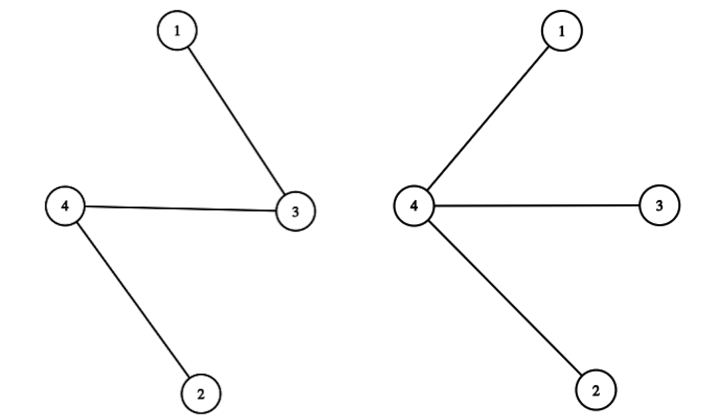시간 제한 메모리 제한 제출 정답 맞은 사람 정답 비율
2 초 512 MB 0 0 0 0.000%

## 문제

In future after the time machine was invented it became easier to learn history. Now you can just go to the corresponding year and watch the events by yourself. Professor uses this device to study the system of Berland roads during the Great Road Transformation.

The transformation took $k$ consectuve years. During these years the system of roads of Berland used to change. There are $n$ cities in the country, numbered from $1$ to $n$. Each year these cities were connected by $n-1$ bidirectional roads, for every pair of cities there was a unique path between them.

Every day Professor chooses two cities $s$ and $f$, travels to every year of the Great Road Transformation, one after another, and makes a trip from $s$ to $f$ in this year, visiting all roads on the path, including $s$ and $f$. After that he wrote down the number of cities that were visited in all of his $k$ trips.

Unfortunately, on the day he had completed his work, having had studied all pairs of cities, he lost all of his records. He only has maps of Berland road system for all $k$ of the Great Road Transformation years.

Help Professor to restore the numbers that he had written down for all possible pairs of cities $s$ and $f$.

## 입력

The first line of input contains two integers $n$ and $k$ --- the number of cities in Berland and the number of years of the Great Road Transformation ($1 \leq n, k \leq 500$).

Then $k$ descriptions of Berland roads systems follow, one for each year, in the following format: $n-1$ lines of the description contains two integers $a$ and $b$ each --- the cities connected by the roads ($1 \leq a, b \leq n$, $a \neq b$).

It is guaranteed that for each of the $k$ years for each pair of cities there is a unique path between them.

## 출력

Output $n$ lines, $n$ integers in each of them. The $j$-th number in the $i$-th line must be the number that Professor wrote down if $s=i$ and $f=j$.

## 예제 입력 1

4 2
1 3
4 2
3 4
1 4
4 3
2 4


## 예제 출력 1

1 3 2 2
3 1 3 2
2 3 1 2
2 2 2 1


## 예제 입력 2

3 3
1 2
2 3
2 3
3 1
3 1
1 2


## 예제 출력 2

1 2 2
2 1 2
2 2 1


## 힌트

There are $4$ cities in Berland in the first sample test. There are $2$ years studied by Professor. The map of the road system for the first year is shown on the left, the map for the second year is shown on the right.Consider the pair of cities $s = 1$ and $f = 2$. Traveling to the first year, Professor visits cities $1$, $2$, $3$, $4$ on his trip. Traveling to the second year, Professor visits cities $1$, $2$, $4$. So there are $3$ cities $1$, $2$, $4$ that will be visited in all his trips, so he writes down the number $3$ for this pair of cities.

Consider the pair of cities $s = 3$ and $f = 1$. Traveling to the first year, Professor visits cities $1$, $3$ on his trip. Traveling to the second year, Professor visits cities $1$, $3$, $4$. So there are $2$ cities $1$, $3$ that will be visited in all his trips, so he writes down the number $2$ for this pair of cities.